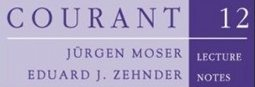## Series Monográficas: Courant lecture notesQA403 V375

Vol. 15 Artin, Emil; Blank, Albert A.
Algebra with Galois theory
QA214 A75

QA184.2 G74 V. II

Vol. 14 Lax, Peter D.
Hyperbolic partial differential equations
QA377 L39

QAQA184.2 G74 V. I

Vol. 12. Moser, Jürgen; Zehnder, Eduard J.
Notes on dynamical systems
QA614.8 M68

Vol. 26 Percus, Jerome kenneth; Childress, Stephen
Mathematical models in developmental biology
QH323.5 Ch54 2015

Supersymmetry for mathematicians : an introduction
QA174.17S86 V37

Vol. 10 Cazenave, Thierry
QC174.26W28 C39

Vol. 23 Percus, Jerome Kenneth
Mathematical methods in immunology
QR182.2M36 P47

Vol. 8 Bogomolov, Fedor; Petrov, Tihomir
Algebraic curves and one-dimensional fields
QA567.2S56 B64

Mathematical methods for analysis of a complex disease
QH323.5 H652

Vol. 7 Varadhan, S. R. S.
Probability theory
QA273 V357

Vol. 6. Nirenberg, Louis; Artino, Ralph A.
Topics in nonlinear functional analysis
QA320 N57

Vol.  20 Esposito, Pierpaolo; Ghoussoub, Nassif; Guo, Yujin
Mathematical analysis of partial differential equations modeling electrostatic MEMS
TK7875 M38

Vol. 19 Childress, Stephen
An introduction to theoretical fluid mechanics
QA901 C425

Vol. 4. Colding, Tobias H.; Minicozzi II, William P.
Minimal Surfaces
This volume has been discontinued

Vol. 2 Shatah, Jalal M. Ihsan; Struwe, Michael
Geometric wave equations
QC174.26W28 S43

Vol. 16 Varadhan, S. R. S.
Stochastic processes
QA273 V36 2007

Vol. 1. Han, Qing; Lin, Fanghua
Elliptic partial differential equations
QA377 H348

### ¿Quién está en línea?

Hay 44 invitados y un miembro en línea

Go to top